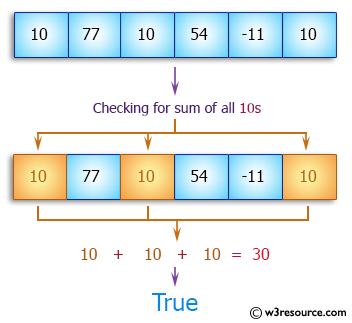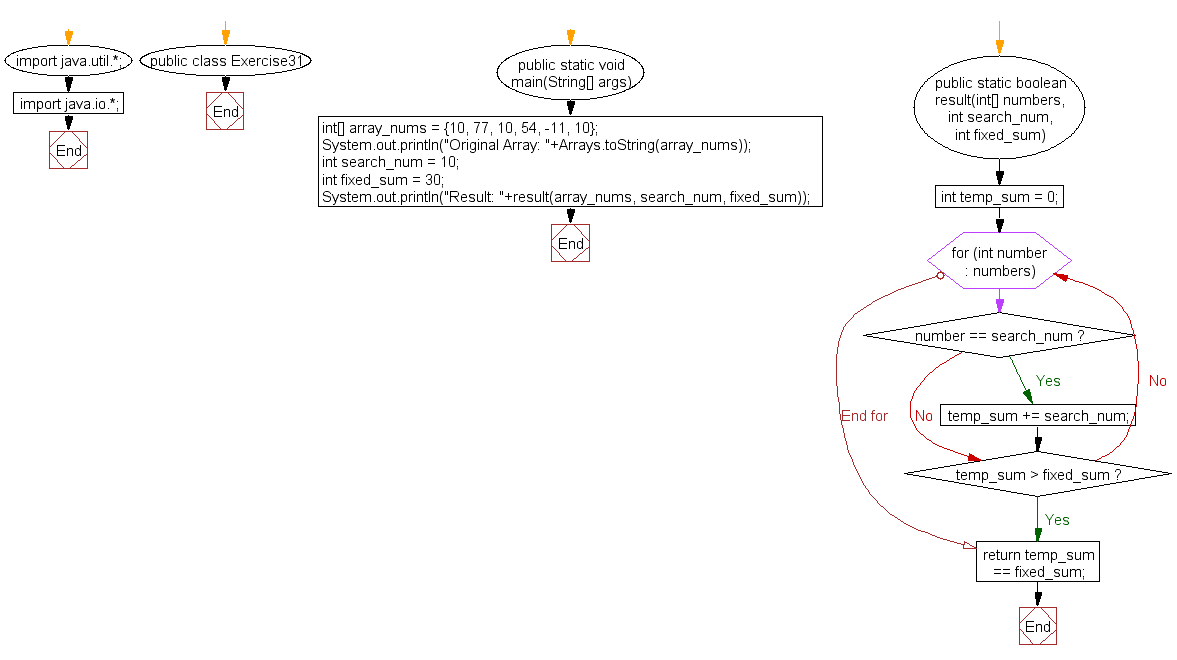﻿ Java: Check the sum of all 10s in the array is exactly 30# Java Array Exercises: Check if the sum of all the 10's in the array is exactly 30

## Java Array: Exercise-31 with Solution

Write a Java program to check if the sum of all the 10's in the array is exactly 30. Return false if the condition does not satisfy, otherwise true.

Pictorial Presentation:Sample Solution:

Java Code:

``````import java.util.*;
import java.io.*;
public class Exercise31 {
public static void main(String[] args)
{
int[] array_nums = {10, 77, 10, 54, -11, 10};
System.out.println("Original Array: "+Arrays.toString(array_nums));
int search_num = 10;
int fixed_sum = 30;

System.out.println("Result: "+result(array_nums, search_num, fixed_sum));
}

public static boolean result(int[] numbers, int search_num, int fixed_sum) {
int temp_sum = 0;
for (int number : numbers) {
if (number == search_num) {
temp_sum += search_num;
}

if (temp_sum > fixed_sum) {
break;
}
}
return temp_sum == fixed_sum;
}
}
```
```

Sample Output:

```
Original Array: [10, 77, 10, 54, -11, 10]
Result: true
```

Flowchart:Visualize Java code execution (Python Tutor):

Java Code Editor:

Improve this sample solution and post your code through Disqus

What is the difficulty level of this exercise?

Test your Programming skills with w3resource's quiz.

﻿

## Java: Tips of the Day

getEnumMap

Converts to enum to Map where key is the name and value is Enum itself.

```public static <E extends Enum<E>> Map<String, E> getEnumMap(final Class<E> enumClass) {
return Arrays.stream(enumClass.getEnumConstants())
.collect(Collectors.toMap(Enum::name, Function.identity()));
}
```

Ref: https://bit.ly/3xXcFZt